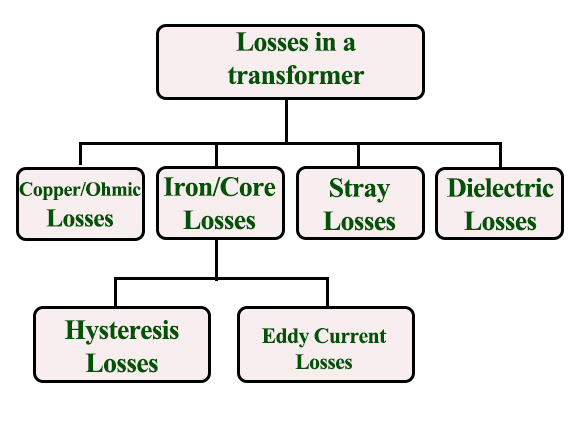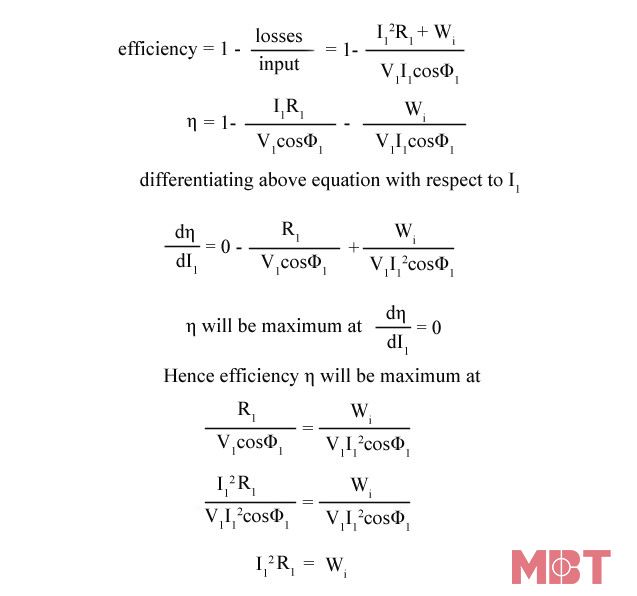# Types of losses in transformers:

An ideal transformer is very efficient, they don’t have energy losses. It means power supplied to the transformer’s input terminal must be equivalent to the power supplied to the transformer’s output one. So the input power and output power in an ideal transformer are equal including zero energy losses.

In reality, both the input and output powers of the transformer will not equal because of electrical losses within the transformer. Because the transformer is a static device, it doesn’t have any movable parts, so we cannot observe mechanical losses but electrical losses will occur like copper and iron. This article discusses an overview of different types of losses in a transformer.

There are different kinds of losses that will be occurred in the transformer such as copper, hysteresis, eddy, iron, stray & dielectric. The copper loss commonly occurs due to the resistance in the transformer winding whereas hysteresis losses will be occurred due to the magnetization change within the core.

1. Core Losses Or Iron Losses

2. Copper Loss

3. Stray Loss

4. Dielectric Loss

5. Measure Efficiency of Transformer

6. Condition For Maximum Efficiency

## 1. Core Losses Or Iron Losses

Eddy current loss and hysteresis loss depend on the magnetic properties of the material used for the construction of the core. So, these losses are also known as core losses or iron losses.

• Hysteresis loss in transformer: The reason is the reversal of magnetization in the transformer core. This loss depends on the volume and grade of the iron, frequency of magnetic reversals and value of flux density. We have the Steinmetz formula:

Wh= ηBmax1.6fV (watts)

Where,   η = Steinmetz hysteresis constant

V = volume of the core in m3

• Eddy current loss in transformer: The  AC current is supplied to the primary winding which sets up alternating magnetizing flux in the transformer. When this flux flow to a secondary winding, it produces induced emf in it. But some part of this flux also gets linked with other conducting parts such as steel core or iron body or the transformer, which will result in induced emf in those parts, causing small circulating current in them. This current is called as eddy current. Due to the current, some energy will be dissipated in the form of heat.

## 2. Copper Loss

The ohmic resistance of the transformer windings creates copper loss.  The copper loss for the primary winding is I12R1 and for the secondary winding is I22R2. Where, I1 and I2 are current in primary and secondary winding respectively, R1 and R2 are the resistances of primary and secondary winding respectively. We can see that Cu loss is proportional to square of the current, and current depends on the load. So that copper loss in transformer varies with the load

3. Stray Loss

The reason for the types of loss is the occurrence of the leakage field. When compared with copper and iron losses, the percentage of stray losses are less, so these losses can be neglected.

## 4. Dielectric Loss

The oil of the transformer is the reason for this loss. Oil in transformer is an insulating material. When the oil in the transformer gets deteriorates then the transformer’s efficiency will be affected.

## 5. Measure Efficiency of Transformer

Like any other electrical machine, the efficiency of a transformer can be defined as the output power divided by the input power. That is efficiency = output / input .

In electrical devices, transformers are the most highly efficient ones. It is due to the fact that most of the transformers have full load efficiency between 95% to 98.5%.

As a transformer being highly efficient, the output value is equivalent to input value, and hence it is impractical to measure the efficiency of the transformer by using output/input.

Another methor to find efficiency of a transformer is using, efficiency = (input - losses) / input = 1 - (losses / input).

6. Condition For Maximum Efficiency

You can see in the below formular:

We have,

Copper loss = I12R1

Iron loss = WiHence, the efficiency of a transformer will be maximum when copper loss and iron losses are equal.

So that, Copper loss = Iron loss.

MBT is a Vietnamese manufacturer of the oil distribution transformers, dry-type transformers, electrical cabinets, and other electrical equipment. Please contact + 84 913 006 538 or email [email protected] for more consultation as well as the quotation of your transformer.

.(+84) 0913.006.538
Skype, Telegram, Wechat ...